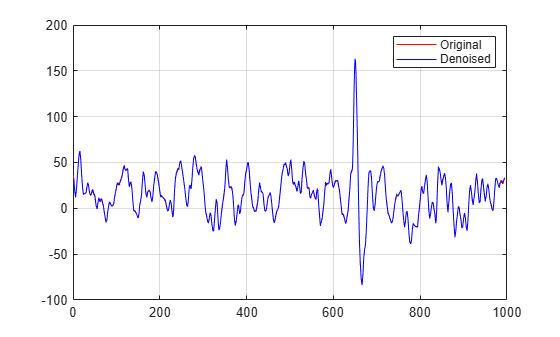# mswden

Multisignal 1-D denoising using wavelets

`mswden` is no longer recommended. Use `wdenoise` instead.

## Syntax

```[XD,DECDEN,THRESH] = mswden('den',...) [XD,THRESH] = mswden('densig',...) [DECDEN,THRESH] = mswden('dendec',...) THRESH = mswden('thr',...) [...] = mswden(OPTION,DIRDEC,X,WNAME,LEV,METH,PARAM) [...] = mswden(...,S_OR_H) [...] = mswden(...,S_OR_H,KEEPAPP) [...] = mswden(...,S_OR_H,KEEPAPP,IDXSIG) ```

## Description

`mswden` computes thresholds and, depending on the selected option, performs denoising of 1-D signals using wavelets.

`[XD,DECDEN,THRESH] = mswden('den',...)` returns a denoised version `XD` of the original multisignal matrix `X`, whose wavelet decomposition structure is `DEC`. The output `XD` is obtained by thresholding the wavelet coefficients, `DECDEN` is the wavelet decomposition associated to `XD` (see `mdwtdec`), and `THRESH` is the matrix of threshold values. The input `METH` is the name of the denoising method and `PARAM` is the associated parameter, if required.

Valid denoising methods `METH` and associated parameters `PARAM` are:

 `'rigrsure'` Principle of Stein's Unbiased Risk `'heursure'` Heuristic variant of the first option `'sqtwolog'` Universal threshold `sqrt(2*log(.))` `'minimaxi'` Minimax thresholding (see `thselect`)

For these methods `PARAM` defines the multiplicative threshold rescaling:

 `'one'` No rescaling `'sln'` Rescaling using a single estimation of level noise based on first level coefficients `'mln'` Rescaling using a level dependent estimation of level noise

### Penalization methods

 `'penal'` Penal `'penalhi'` Penal high, `2.5` ℜ≤ `PARAM` ℜ≤ `10` `'penalme'` Penal medium, `1.5` ℜ≤ `PARAM` ℜ≤ `2.5` `'penallo'` Penal low, `1` ℜ≤ `PARAM` ℜ≤ `2`

`PARAM` is a sparsity parameter, and it should be such that: `1``PARAM``10`. For `penal` method, no control is done.

### Manual method

 `'man_thr'` Manual method

`PARAM` is an `NbSIG`-by-`NbLEV` matrix or `NbSIG`-by-(`NbLEV+1`) matrix such that:

• `PARAM(i,j)` is the threshold for the detail coefficients of level `j` for the ith signal (`1``j``NbLEV`).

• `PARAM(i,NbLEV+1)` is the threshold for the approximation coefficients for the `i`th signal (if `KEEPAPP` is `0`).

where `NbSIG` is the number of signals and `NbLEV` the number of levels of decomposition.

Instead of the `'den'` input `OPTION`, you can use `'densig'`, `'dendec'` or `'thr'` `OPTION` to select output arguments:

`[XD,THRESH] = mswden('densig',...)` or ```[DECDEN,THRESH] = mswden('dendec',...)```

`THRESH = mswden('thr',...)` returns the computed thresholds, but denoising is not performed.

The decomposition structure input argument `DEC` can be replaced by four arguments: `DIRDEC`, `X`, `WNAME` and `LEV`.

`[...] = mswden(OPTION,DIRDEC,X,WNAME,LEV,METH,PARAM)` before performing a denoising or computing thresholds, the multisignal matrix `X` is decomposed at level `LEV` using the wavelet `WNAME`, in the direction `DIRDEC`.

You can use three more optional inputs:

`[...] = mswden(...,S_OR_H)` or
```[...] = mswden(...,S_OR_H,KEEPAPP)``` or
```[...] = mswden(...,S_OR_H,KEEPAPP,IDXSIG)```

• `S_OR_H ('s' or 'h') ` stands for soft or hard thresholding (see `mswthresh` for more details).

• `KEEPAPP (true or false)` indicates whether to keep approximation coefficients (`true`) or not (`false`).

• `IDXSIG` is a vector that contains the indices of the initial signals, or `'all'`.

The defaults are, respectively, `'h'`, `false` and `'all'`.

## Examples

collapse all

Load the 23 channel EEG data `Espiga3` . The channels are arranged column-wise. The data is sampled at 200 Hz.

`load Espiga3`

Perform a decomposition at level 2 using the `db2` wavelet.

`dec = mdwtdec('c',Espiga3,2,'db2')`
```dec = struct with fields: dirDec: 'c' level: 2 wname: 'db2' dwtFilters: [1x1 struct] dwtEXTM: 'sym' dwtShift: 0 dataSize: [995 23] ca: [251x23 double] cd: {[499x23 double] [251x23 double]} ```

Denoise the signals using the universal method of thresholding (`sqtwolog`) and the `'sln'` threshold rescaling (with a single estimation of level noise, based on the first level coefficients).

`[xd,decden,thresh] = mswden('den',dec,'sqtwolog','sln');`

Plot an original signal, and the corresponding denoised signal.

```idxA = 3; plot(Espiga3(:,idxA),'r') hold on plot(xd(:,idxA),'b') grid on legend('Original','Denoised')```Birgé, L., and P. Massart. “From Model Selection to Adaptive Estimation.” Festschrift for Lucien Le Cam: Research Papers in Probability and Statistics (E. Torgersen, D. Pollard, and G. Yang, eds.). New York: Springer-Verlag, 1997, pp. 55–88.

 DeVore, R. A., B. Jawerth, and B. J. Lucier. “Image Compression Through Wavelet Transform Coding.” IEEE Transactions on Information Theory. Vol. 38, Number 2, 1992, pp. 719–746.

 Donoho, D. L. “Progress in Wavelet Analysis and WVD: A Ten Minute Tour.” Progress in Wavelet Analysis and Applications (Y. Meyer, and S. Roques, eds.). Gif-sur-Yvette: Editions Frontières, 1993.

 Donoho, D. L., and I. M. Johnstone. “Ideal Spatial Adaptation by Wavelet Shrinkage.” Biometrika. Vol. 81, pp. 425–455, 1994.

 Donoho, D. L., I. M. Johnstone, G. Kerkyacharian, and D. Picard. “Wavelet Shrinkage: Asymptopia?” Journal of the Royal Statistical Society, series B, Vol. 57, No. 2, pp. 301–369, 1995.

 Donoho, D. L., and I. M. Johnstone. “Ideal denoising in an orthonormal basis chosen from a library of bases.” C. R. Acad. Sci. Paris, Ser. I, Vol. 319, pp. 1317–1322, 1994.

 Donoho, D. L. “De-noising by Soft-Thresholding.” IEEE Transactions on Information Theory. Vol. 42, Number 3, pp. 613–627, 1995.

 Mesa, Hector. “Adapted Wavelets for Pattern Detection.” In Progress in Pattern Recognition, Image Analysis and Applications, edited by Alberto Sanfeliu and Manuel Lazo Cortés, 3773:933–44. Berlin, Heidelberg: Springer Berlin Heidelberg, 2005. https://doi.org/10.1007/11578079_96.

## SupportGet trial now